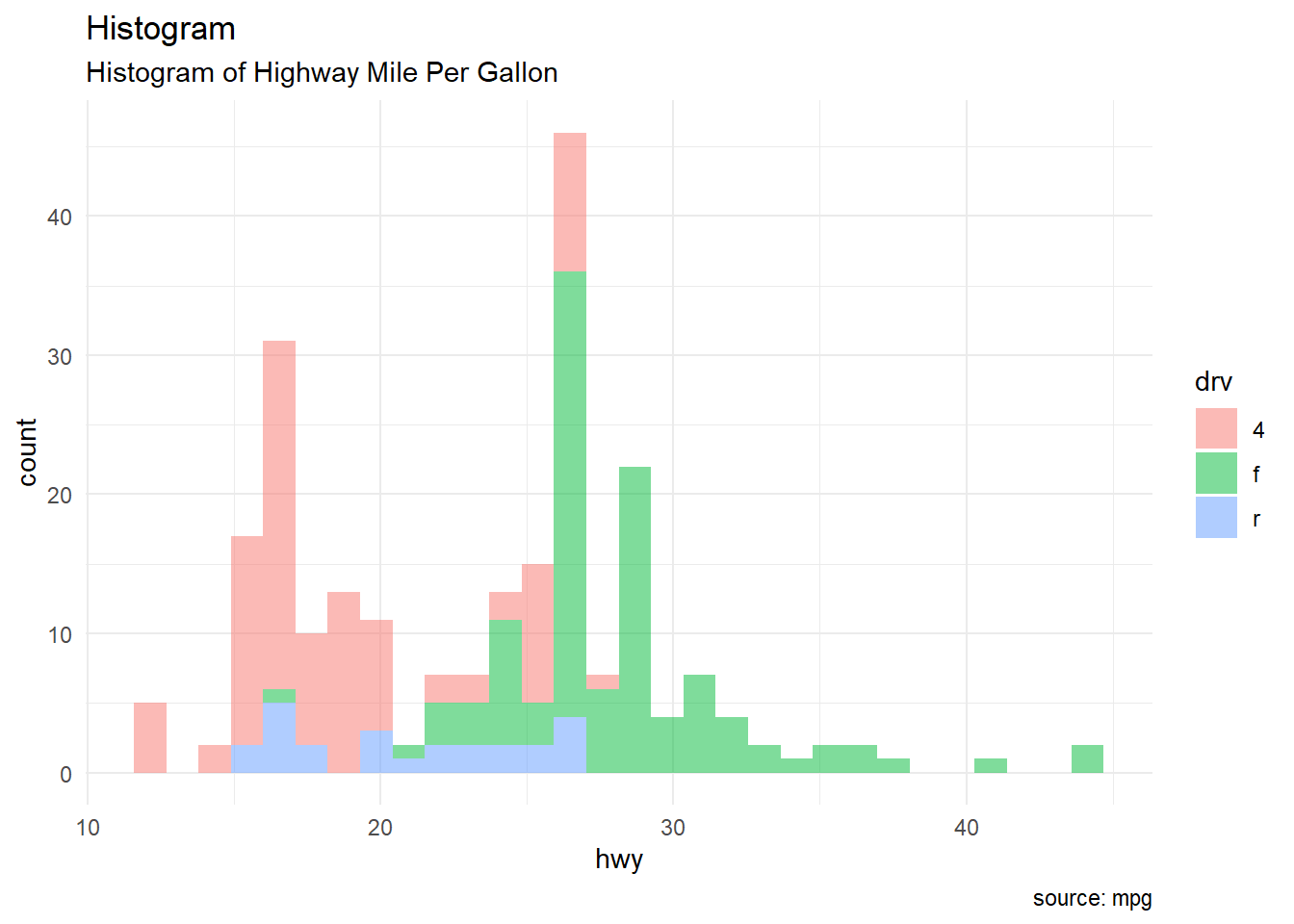# Chapter1 Problem 1

Using the mpg dataset in the ggplot2 package, replicate the plot below using the following settings:

• Set alpha = 0.5 for the width of bars
• Use theme_minimal()

``````library(ggplot2)
``## `stat_bin()` using `bins = 30`. Pick better value with `binwidth`.``